Planet Quantum News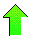Contents News View

 Mar 14, 2017 - Mathieu Function Eigenvalues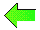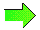Greetings PLANETQUANTUM.COM visitor. Today we discuss Mathieu function eigenvalue formulas.

## Mathieu Functions

Mathieu functions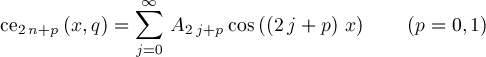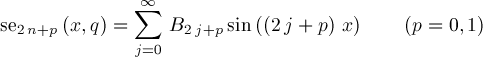are periodic solutions of differential equation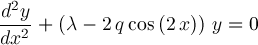having periods π and 2π for p=0 and p=1 respectively. Even solutions ceν(x,q) (ν=0,1,2,...) and odd solutions seν(x,q) (ν=1,2,...) correspond to countably many eigenvalues λ(q)=aν(q) and bν(q) respectively. Additionally, aν(q) and bν(q)=ν2+O(q) for q near 0 . The coefficients Aj and Bj satisfy recurrences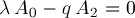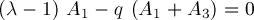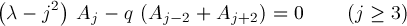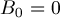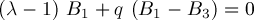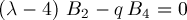It is also usual to apply one of the normalization conditions## Continued Fractions Involving λ

Let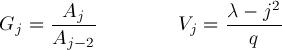The Aj recurrences of the preceding section lead toFurther considerations get Wang & Guo p621 formula (3)Letting ν=0 and k=0 gets Wang & Guo p621 formula (4)for even ν period π solutions. Another observation gets Wang & Guo p621 formula (5)We like to use formula (5) to compute b2(q). From formula (5), we see b2(q)=4+O(q^2) for small q. Substituting λ=4+O(q^2) into the RHS of formula (5) gets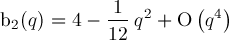Iterating gets better approximations: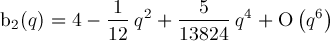The procedure is automated in MathieuFormulaB2.q.txt. Using MathieuFormulaB2.q.txt and similar code, we can derive formulas for aν(q) and bν(q) when ν≤10 .

We use Wang & Guo p621 (3), p623 (8), p623 (11), p621 (5), and p627 (7) to compute aν(q) for even ν≥0, a1(q) and b1(q), aν(q) and bν(q) for odd ν≥3, b2(q), and bν(q) for even ν≥4, respectively. For brevity, we omit detailing these cases. Some typos discovered on Wang & Guo p623 are mentioned in our MathieuFormulaB2.q.txt code comments.

## Formulas for aν(q) and bν(q) when ν≤10

The following formulas we've generated may be compared with Abramowitz & Stegun p724 20.2.25, Wang & Guo pp628-629 (4), and NIST 28.6.1-13 (see References):

Formula for a0(q):Formula for a1(q):Formula b1(q)=a1(-q).

Formula for a2(q):Formula for b2(q):Formula for a3(q):Formula b3(q)=a3(-q).

Formula for a4(q):Formula for b4(q):Formula for a5(q):Formula b5(q)=a5(-q).

Formula for a6(q):Formula for b6(q):Formula for a7(q):Formula b7(q)=a7(-q).

Formula for a8(q):Formula for b8(q):Formula for a9(q):Formula b9(q)=a9(-q).

Formula for a10(q):Formula for b10(q):We've placed these formulas in text form in News170314.q.txt .

## Formula for aν(q) and bν(q) when ν≥11

We can also generate general formulas for symbolic ν≥N where N can take on various concrete values. The next formula may be compared with Abramowitz & Stegun p724 20.2.26, Wang & Guo p629 (5), and NIST 28.6.14 (see References). The formula does not imply aν(q)=bν(q), just aν(q)=bν(q)+O(q11).

Formula for aν(q) and bν(q) when ν≥11:## _FloatMathieuEigenB2

Let's describe a function FloatMathieuEigenB2 for computing b2(q) using continued fraction convergents. In general, numerators pn and denominators qn of successive convergents pn/qn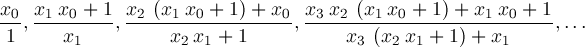of a continued fractionsatisfy recurrence relations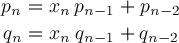Lettinggets us a numerical implementation of Wang & Guo p621 formula (5). Starting with small q and initial approximation λ=4 for b2(q), repeatedly applying Wang & Guo p621 formula (5) gives increasingly better approximations converging to b2(q). We've implemented this theory by two functions _FloatMathieuEigenB2 and _FloatMathieuEigenB2Step in FloatMathieuEigenB2.q.txt. Let's try it out.

>(MathieuB 2 0.5)
3.979189215751358
>(Eval (Subst 'q 0.5 b2))
3.979189215751358
>(MathieuB 2 5.)
2.099460445486666
>(Eval (Subst 'q 5. b2))
2.099593807819844
>(SetPrecision 3000)
3000
>(MathieuB 2 5.)
2.09946044548666536395565456881965731303214394331170320017824203\
0096910666016096613169463818290910455650222866107481368603826818\
2200885982391039191016922951691897213446342709546044123319893224\
4884779534875094432194561990996001898932313100168622128963566755\
2444799315475114167572014411096483980218759337156610966126207955\
3363964379976071374935610048542924550102678732839474622165336383\
0067067482178047952633487575457431874307824633234823151409436199\
8786873010088485494067402909622972233594584633679500216364218960\
6063396454077401028375665634968944833812045262700930370779049038\
3793978604457590588170909146959619599196244413809076892648159886\
1957883688707095515970390508393946463490626842421943592404583448\
0864433781550131376210228929628763001849675083051023805804201152\
1601438315610853345308704126733916766040169171274208341403528809\
6696506658587696813249633857942585672721678187404117233059374475\
73677448

Abramowitz & Stegun p749 (Table 20.1) gives b2(5.)=2.09946045  .

## _FloatMathieuSe

Let's use the eigenvalue (MathieuB 2 0.5) just computed to compute (MathieuSe 2 1. 0.5) . Here is what we get:

>(MathieuSe 2 1. 0.5)
.93979586513485
>(SetPrecision 3000)
3000
>(MathieuSe 2 1. 0.5)
.939795865134850041321678599481074546543262353406214114560268136\
8196540239619884690957002732827552779069837332177149621585041116\
8976798645480024426901215984830593452983662685294768303657473252\
0860218992214922224741591366430370338480542298581534202903552027\
6988366636597377708410528576564072790075734001941052307653142850\
5642983542365998686172741582862899971992421575398112553164272012\
9006459367958829277619106068329006366963204110893091488526396115\
0577894100844961888405895444155032836355894314832703732934114758\
6294014368835133940117757556488032470930881996175776298583883469\
6472818571577806083454535926227921179828255195281776622581385055\
7925365840943668310034904112549202367394490044685600574045069700\
1583455641555883218733669121664335272259948510014873224908867258\
7848789167852035522487911323779219510079541420879518183739450766\
7322680801736431603890146945988466236317979940666868486905042518\
39762175

We put additional details of this calculation and some source code in MathieuSeTrace.txt and FloatMathieuSe.q.txt

## Conclusion

We've discussed Mathieu functions ceν(x,q) and seν(x,q), continued fractions involving Mathieu function eigenvalues λ(q)=aν(q) and bν(q), formulas for aν(q) and bν(q) for small q, a numerical method for computing eigenvalues via continued fraction convergents, and illustrating calculations of (MathieuB 2 0.5), (MathieuB 2 5.), and (MathieuSe 2 1. 0.5).

We might cover calculating Mathieu Functions for large q in a future post.

## References

Arthur Erdélyi, ed., (1955) CHAPTER XVI MATHIEU FUNCTIONS, SPHEROIDAL, AND ELLIPSOIDAL WAVE FUNCTIONS, Higher Transcendental Functions, Volume III, Robert E. Krieger Publishing Company, Malabar, Florida.

Milton Abramowitz and Irene A. Stegun, eds. (1965) 20. Mathieu Functions, HANDBOOK OF MATHEMATICAL FUNCTIONS with Formulas, Graphs, and Mathematical Tables, Dover Publications, Inc.

F. W. J. Olver, A. B. Olde Daalhuis, D. W. Lozier, B. I. Schneider, R. F. Boisvert, C. W. Clark, B. R. Miller, and B. V. Saunders, eds. (2016) 28.6 Expansions for Small q, NIST Digital Library of Mathematical Functions, Release 1.0.14 of 2016-12-21.

Z. X. Wang and D. R. Guo, (1989) Chapter 12: MATHIEU FUNCTIONS, Special Functions, World Scientific Publishing Co Pte Ltd.

Wikipedia Contributors, (2017) Continued fraction, Infinite continued fractions and convergents, Wikipedia, The Free Encyclopedia.

Wikipedia Contributors, (2017) Mathieu function, Wikipedia, The Free Encyclopedia.